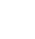# Import multiple csv files to 1 column in 1 sheet

``` Application.ScreenUpdating = False```

``` Worksheets("Sheet1").Cells.ClearContents Dim qry As QueryTable Dim FilNams As Variant Dim FilNamCntr As Long Dim strQryName As String Dim LastRow As Long Dim ContainerWB As Workbook Dim msgString As String Dim rng As Range Dim iCol As Integer Dim lastCell As Integer FilNams = Application.GetOpenFilename(FileFilter:="Text Files (*.csv),*.csv", _ Title:="Select Textfile to Import", _ MultiSelect:=True) 'Check to see if any files were selected If TypeName(FilNams) = "Boolean" Then MsgBox "No Files Selected. Exiting Program." Exit Sub Else 'msgString = Join(FilNams, vbCr) 'MsgBox "FilNams is: " & msgString End If For FilNamCntr = LBound(FilNams) To UBound(FilNams) FilNams(FilNamCntr) = "TEXT;" & FilNams(FilNamCntr) Next FilNamCntr 'msgString = Join(FilNams, vbCr) 'MsgBox "FilNams is: " & msgString For FilNamCntr = LBound(FilNams) To UBound(FilNams) With ActiveSheet On Error GoTo ErrorCatch: 'Append to previous data, if applicable If .Range("A" & Rows.Count).End(xlUp).Row = 1 Then LastRow = 1 Else LastRow = .Range("A" & Rows.Count).End(xlUp).Row + 1 End If 'MsgBox "LastRow value is:" & LastRow 'verification test Set qry = .QueryTables.Add(Connection:=FilNams(FilNamCntr), _ Destination:=.Range("A" & LastRow)) With qry .Name = "Filename" .FieldNames = False .RowNumbers = False .FillAdjacentFormulas = False .PreserveFormatting = True .RefreshOnFileOpen = False .RefreshStyle = xlInsertDeleteCells .SavePassword = False .SaveData = True .AdjustColumnWidth = True .RefreshPeriod = 0 .TextFilePromptOnRefresh = False .TextFilePlatform = xlWindows .TextFileStartRow = 1 .TextFileParseType = xlFixedWidth .TextFileTextQualifier = xlNone .TextFileConsecutiveDelimiter = False .TextFileTabDelimiter = False .TextFileSemicolonDelimiter = False .TextFileCommaDelimiter = True .TextFileSpaceDelimiter = False .TextFileColumnDataTypes = Array(1, 1, 1, 1, 1, 1, 1, 1, 1) .Refresh BackgroundQuery:=False End With End With Next FilNamCntr For c = 500 To 1 Step -1 If ActiveSheet.Cells(1, c) = "" Then ActiveSheet.Cells(1, c).EntireColumn.Delete shift:=xlLeft End If Next c Set rng = ActiveCell.CurrentRegion lastCell = rng.Columns(1).Rows.Count + 1 For iCol = 2 To rng.Columns.Count Range(Cells(1, iCol), Cells(rng.Columns(iCol).Rows.Count, iCol)).Cut ActiveSheet.Paste Destination:=Cells(lastCell, 1) lastCell = lastCell + rng.Columns(iCol).Rows.Count Next iCol ```

```Exit Sub ErrorCatch: MsgBox "Unexpected Error. Type: " & Err.Description ```

### One thought on “Import multiple csv files to 1 column in 1 sheet”

1.Nick says:

Example code updated 23/12/2015 to reset the process used to determine the number of columns that are used, so that where only 2 columns are present, they are both considered.